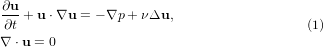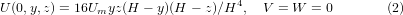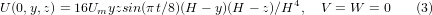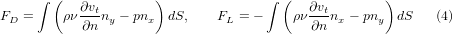### Benchmark configurations

The solvers are tested in two benchmark configurations with an incompressible Newtonian fluid whose kinematic viscosity (ν) is equal to 10-3 m2/s and for which the conservation equations of mass and momentum are written as follows,$\begin{equation}\begin{split} &\frac{\partial \mathbf{u}}{\partial t} +\mathbf{u}\cdot\nabla \mathbf{u}=-\nabla p +\nu\Delta\mathbf{u},\\ &\nabla\cdot\mathbf{u}=0 \end{split} \label{NS} \end{equation}$

The Reynolds number is defined as Re=UD/ν where U is the mean velocity of the imposed parabolic profile on the inflow boundary and D is the diameter of the cylinder. The benchmark geometry and the corresponding 2D mesh at the coarsest level are shown in the figure below. The 3D mesh is obtained by extruding the 2D mesh in the z direction with 4 layers of cells, however the first level mesh for the computations is obtained by two successive refinements via connecting opposite midpoints of the the coarsest mesh which yields a mesh of 6144 cells. Our preliminary studies showed that this mesh offers a good balance between accuracy and computational cost.The software tools employ different numerical approaches to the discretization in space which leads to different numbers of degrees of freedom (DOF) for the same problem. In the case of OpenFOAM and CFX, the numbers of DOF are comparable while FeatFlow has a greater number of DOF for the same mesh due to a high order finite element approximation. Therefore, while comparing the results, reader should keep in mind that for the same computational mesh, FeatFlow has approximately as many DOF as the others have at one level finer grid. The number of DOF is always proportional to number of cells (equivalent to number of vertices for hexahedral meshes with large number of cells) in all CFD packages. The numbers of DOF are presented with respect to the number of cells of the corresponding mesh levels in the table below.

• ndof = 3 · nvt for velocity and ndof = nvt for pressure in case of CFX.
• ndof = 3 · nel for velocity and ndof = nel for pressure in case of OpenFOAM.
• ndof = 24 · nvt for velocity and ndof = 4 · nel for pressure in case of FeatFlow.

where ndof, nvt and nel denote the number of DOF, the number of vertices and the number of cells, respectively.

Number of unknowns
Levels # of cells Software # of DOF u # of DOF P total # of DOF
CFX 21828 7276 29104
L1 6144 OF 18423 6144 24567
FeatFlow 174624 24576 199200
CFX 160776 53592 214368
L2 49152 OF 147456 49152 196608
FeatFlow 1286208 196608 1482816
CFX 1232400 410800 1643200
L3 393216 OF 1179648 393216 1572864
FeatFlow 9859200 1572864 11432064
CFX 9647136 3215712 12862848
L4 3145728 OF 9437184 3145728 12582912
FeatFlow 77177104 12582912 89760016

The first benchmark problem is at Re=20 and has been studied numerically by many research groups, and very accurate results have been presented. The second benchmark problem is unsteady, with a time-varying inflow profile which is very challenging and has not been rigorously studied, consequently the results have not yet been precisely determined. For the benchmark problems, no-slip boundary condition is employed on the walls and natural do-nothing boundary conditions are imposed at the outflow plane. The inflow conditions are set with U_m=0.45 m/s and U_m=2.25 m/s for the first and the second benchmark problem with the parabolic velocity profiles from equations (2) and (3), respectively,$\setcounter{equation}{1} \begin{equation} U(0,y,z) = 16U_myz(H-y)(H-z)/H^4,\quad V=W=0 \label{parabolicProfile} \end{equation}$$\setcounter{equation}{2} \begin{equation} U(0,y,z) = 16 U_m y z sin(\pi t/8) (H-y) (H-z)/H^4,\quad V=W=0 \label{parabolicProfileT} \end{equation}$

where FD and FL are defined as,$\setcounter{equation}{3} \begin{equation} F_D = \int \left( \rho \nu \frac{\partial v_t}{\partial n}n_y - p n_x\right) dS , \qquad F_L = -\int \left( \rho \nu \frac{\partial v_t}{\partial n}n_x - p n_y\right) dS \end{equation}$

with the following notations: surface of cylinder S, normal vector n on S with x- and y- component nx and ny, tangential velocity vt on S and tangent vector t=(ny,-nx,0).